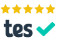# Edexcel A level maths mechanics 9MA0 SAM: interactive! (with mouse)

### Hover the mouse over a vector for guidance.ARecommended Resource

Main page - loads of interactive A level mechanics

7.(a) First always collect all the forces acting, and show their directions. Here they are W, Fmax and R, and we can see that only the friction and a component of the weight are going to act parallel to the slope.

Now right click the grey circle which will appear when you hover near the base of the W vector, so that this arrow disappears. Then hover the mouse near the join of the three vectors bottom right (the one with a red resultant of magnitude mg) - so that a grey sausage shape (not a circle) is visible. Then left click and drag the whole shape so that it replaces the missing W vector to represent the weight of the particle. Now click on the grey circle at the join and drag to play around. For example drag at the join (grey circle) so that the initially vertical red (resultant) arrow is horizontal. Try to see how the "big and little" angles in the right triangle in the diagram show you how to draw a vector parallelogram which is correct just because "big and little" correspond appropriately.

Now press control-Z until the display is back to how it was. See how Newton's second law (ΣF = ma) means the particle will be moving up the slope with a "negative" force (with a negative rate of increase in momentum, so we can represent the force as down the slope) equal to the sum total of the two retarding forces: friction and three fifths of the weight. (Three fifths because of the 3-4-5 triangle. Click near the end of a vector to get dotted lines completing the triangles, which may help to see the trigonometry.)

Solve for μ as shown.

(b) When there is no motion upwards, friction will begin to act against the downward weight, and reach the same maximum as before but now acting up the slope. So just compare the magnitudes of weight and friction.

Your browser does not support the HTML5 canvas tag.

load a saved work file or a background image file:

8.

(a) If in doubt, sketch a velocity-time graph for each of the horizontal and vertical components. Calculate the change in v over the change in t, for each component of the acceleration.

(b) For each component, you could form a general expression for v in terms of t, then integrate. But of course, you know that constant acceleration means you can use s = ut + ½2, as shown.

(c) The 2 expressions you just found will be equal when the position vector is pointing NE, and you can solve for t. With the shift button held down, left click and drag on the tip of the resultant position vector, to see it and its components varying with t, following the path coloured blue.

(d) Similarly for the 2 velocity expressions (which you may now need to form if you used the suvat for part (b)). Again, do shift-drag to see the direction of the velocity change with time t.

Your browser does not support the HTML5 canvas tag.

load a saved work file or a background image file:

9.

(a) You can always assume the reaction against a wall or floor to be the resultant of a perpendicular reaction and a parallel friction. In this case the wall is smooth, so the reaction is purely horizontal.

Hover the mouse near the join of the vector parallelogram top centre (the one with a red resultant of magnitude RB) - so that a grey sausage shape (not a circle) is visible. Then left click and drag the whole shape so that it acts (pulls) at B. Try to see how the "big and little" angles in the right triangle in the diagram show you how to draw a vector parallelogram which is correct just because "big and little" correspond appropriately. (Even though the diagram isn't pronouncing big and little nearly as much as it should for a ratio of 5 to 2.)

Do similarly for the two weight parallelograms. Press control-z to return to the initial state. Now drag the 3 rotatable moments (again, hover near but not quite on the join) so that their join is on A. See how each of them corresponds to a component of either RB or a weight, each multiplied by the appropriate measure of distance from A. Rotate them (click and drag the grey circle half way along) and sense how equilibrium means that the clockwise one of the 3 moments will equal the sum of the 2 anticlockwise ones. So you can form an equation and solve for RB, as shown.

(b) You only need to resolve horizontally here. The forces involved are F, P and RB. Either F is acting in concert with P against RB, in which case P can be as low as... ? Or else F is acting in concert with RB against P, in which case P could be as large as... ?

(c) Assume they stand on the very bottom of the ladder, and you can see that their weight is irrelevant to the moments about A, just as were the friction and reaction at A, earlier. So maximum friction at A is now increased, and the minimum value of P needed to maintain equilibrium is also reduced.

Your browser does not support the HTML5 canvas tag.

load a saved work file or a background image file:

Main page - more interactive A level mechanics

ARecommended Resource
Garden City Maths - Online tuition!Today! Just open a meeting in Zoom and send me an invite by email (below). Or email me.
Balloon Calculus ... Don't integrate - balloontegrate!

email: tom@ballooncalculus.org.